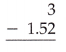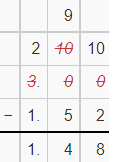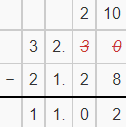# McGraw Hill Math Grade 6 Lesson 11.2 Answer Key Subtracting Decimals

Practice questions available in McGraw Hill Math Grade 6 Answer Key PDF Lesson 11.2 Subtracting Decimals will engage students and is a great way of informal assessment.

Exercises Subtract

Question 1.By subtracting 23.01 and 22.99 we get 0.02

Question 2.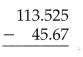By subtracting 113.525 and 45.67 we get 67.855

Question 3.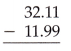By subtracting 32.11 and 11.99 we get 20.12

Question 4.By subtracting 10.25 and 0.75 we get 9.50

Question 5.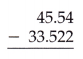By subtracting 45.54 and 33.522 we get 12.018

Question 6.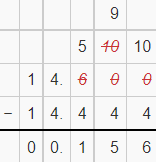By subtracting 14.6 and 14.444 we get 0.156

Question 7.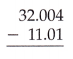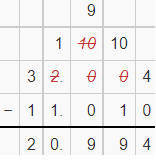By subtracting 32.004 and 11.01 we get 20.994

Question 8.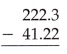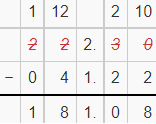By subtracting 222.3 and 41.22 we get 181.08

Question 9.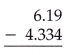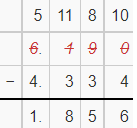By subtracting 6.19 and 4.334 we get 1.856

Question 10.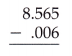By subtracting 5.565 and 0.006 we get 8.559

Question 11.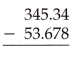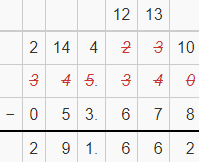By subtracting 345.34 and 53.678 we get 291.662

Question 12.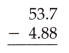By subtracting 53.7 and 4.88 we get 48.82

Question 13.By subtracting 2.398 and 1.998 we get 0.4

Question 14.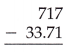By subtracting 717 and 33.71 we get 683.29

Question 15.